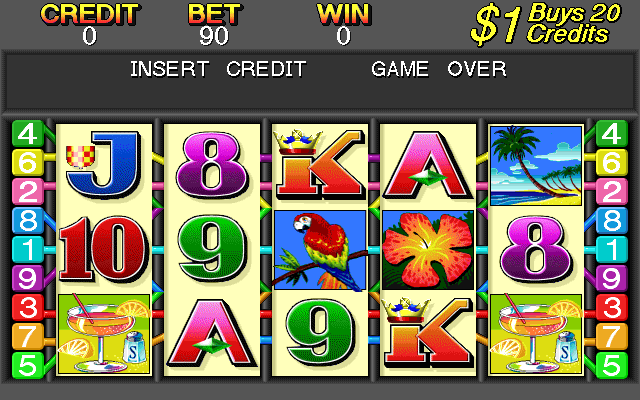# Tropical Delight

## Video Slot Machine published 25 years ago by Aristocrat Leisure Industries Pty

#### Listed in MAMETropical Delight © 1997 Aristocrat Leisure Industries Pty, Ltd.

A 5-reel video slot machine.

TECHNICAL

Aristocrat MK5 hardware.

TRIVIA

Tropical Delight's theme was reused in the game "Tahiti Magic".

SCORING

All pays are left to right including scattered Parrots. Girl substitutes for all symbols except Parrot.

Paytable:

Girl : 1 = 2, 2 = 25, 3 = 300, 4 = 2000, 5 = 5000
Flower : 1 = 1, 2 = 5, 3 = 120, 4 = 500, 5 = 2500
Dolphin : 1 = 1, 2 = 5, 3 = 120, 4 = 500, 5 = 2500
Tree : 2 = 5, 3 = 100, 4 = 250, 5 = 1000
Sunset : 2 = 5, 3 = 100, 4 = 250, 5 = 1000
Drink : 3 = 40, 4 = 75, 5 = 500
Ace : 3 = 40, 4 = 75, 5 = 500
King : 3 = 10, 4 = 50, 5 = 200
Queen : 3 = 10, 4 = 50, 5 = 200
Jack : 3 = 10, 4 = 40, 5 = 150
Ten : 3 = 10, 4 = 40, 5 = 150
Nine : 2 = 2, 3 = 5, 4 = 20, 5 = 120
Eight : 3 = 5, 4 = 20, 5 = 100
Seven : 3 = 5, 4 = 20, 5 = 100
Parrot : 2 = 2, 3 = 5, 4 = 20, 5 = 50

TIPS AND TRICKS

* Feature : Any left to right scatter win triggers 3 free spins.
Initial scatter win is held during feature.
During feature scatters pay any.
Any additional Parrots spun up are held and 3 spins recommence.
When a 5-of-a-kind Parrot win occurs the 5x Parrot win is replayed 3 times.

* It is not possible to get a line win during the Parrot spins due to the location of the symbols.

SERIES
SOURCES

Slot's picture.